# Exponential Equations

• Introduction

• Properties of Powers

• 25 Resolved Exponential Equations step by step

## Introduction

An exponential equation is one that has exponential expressions, in other words, powers that have in their exponent expressions with the unknown factor x.

In this section, we will resolve the exponential equations without using logarithms. This method of resolution consists in reaching an equality of the exponentials with the same base in order to equal the exponents.

For example:

$$3^{2x} = 3^6$$

Obviously, the value that x has to take for the equality to be true is 3.

In order to achieve this type of expressions we have to factorize, express all the numbers in the form of powers, apply the properties of powers and write roots as powers.

Sometimes we will need to make a change of variable to transform the equation in a quadratic one.

We can also resolve using logarithms, but we will leave this type of procedures for more difficult equations with different bases in the exponential expressions, making it impossible to use the previous method of equalizing.

For example,

$$3^{x+3} = 5^x$$

which has a real solution, using logarithms of,

$$x = \frac{3 ln(3)}{ln\left(\frac{5}{3}\right)}$$

## Before we start...let's remember the properties of powers

 Product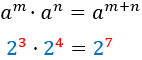Power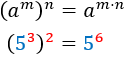Quotient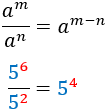Negative exponent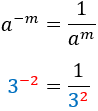Inverse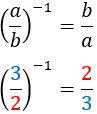Inverse of inverse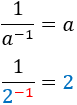# Solved Exponential Equations

Equation 1Show solution

Equation 2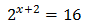Show solution

Equation 3Show solution

Equation 4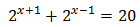Show solution

Equation 5Show solution

Equation 6Show solution

Equation 7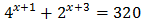Show solution

Equation 8Show solution

Equation 9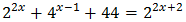Show solution

Equation 10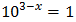Show solution

Equation 11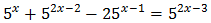Show solution

Equation 12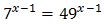Show solution

Equation 13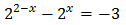Show solution

Equation 14Show solution

Equation 15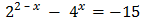Show solution

Equation 16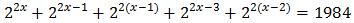Show solution

Equation 17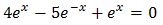Show solution

Equation 18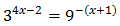Show solution

Equation 19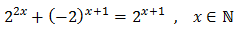Show solution

Equation 20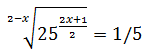Show solution

Equation 21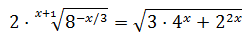Show solution

Equation 22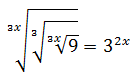Show solution

Equation 23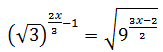Show solution

Equation 24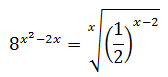Show solution

Equation 25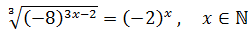Show solution